Calibration of a rotary spreader is a two-part process that requires:

1. Measurement of the swath width.
2. Determination of the application rate.

## Measurement of the Swath Width and Distribution Pattern

Check the distribution pattern of rotary spreaders frequently so that you will not end up applying too much product to some areas and not enough to others even if the application rate is correct. A good way to check the distribution pattern is to use a row of shallow boxes, trays, or pans in a line perpendicular to the direction of travel (see figure below).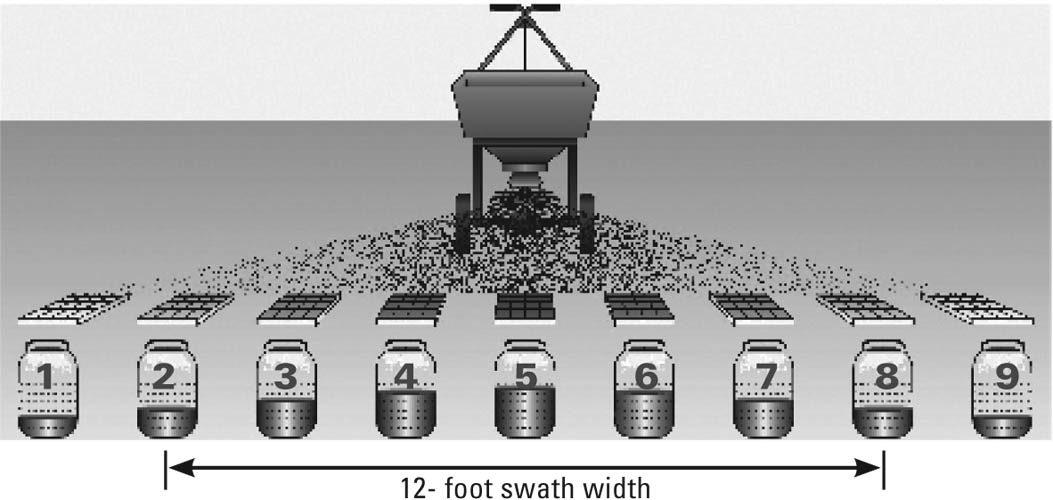Use boxes that are identical in size, 1 to 2 inches deep, with an area of at least 1 square foot. You will need an odd number (7 or 9 will usually work well).

Step 1.  Place one box in the center and space the remaining boxes on 2-foot centers to either side of the center box as shown in the figure.

Step 2.  Pour some product into the spreader and follow the setting recommendation on the label. Make 3 passes over the boxes—always from the same direction.

Step 3.  Record the weight of granules collected from each box, or pour granules into a jar marked with the box number. When you line up the jars in the same order as the collection boxes, you will see the distribution pattern. The center jar should have the most product, with amounts tapering off evenly on each side. If the pattern is not even on each side, follow the manual instructions for adjusting the spreader and then repeat Steps 2 and 3 until the spreader is applying material in the most uniform pattern possible.

Step 4.  Find the jars to each side that have half the amount in the center jar. The distance between the boxes represented by these bottles is the effective swath width.

#### Example

Determining effective swath width for two rotary spreaders.

 Spreader #1 Spreader #2 Depth of granules in center jar = 2 inches Depth of granules in center jar = 3 inches Depth of granules in jars 2 and 8 = 1 inch Depth of granules in jars 2 and 8 = 1 inch Swath width = 12 ft. (6 ft. to each side of center) Depth of granules in jars 3 and 7 = 2 inches Swath width = 10 feet (5 feet to each side)

## Determination of the Application Rate

Because the distribution pattern of a rotary spreader is rarely uniform, it is best to make two passes over a treatment site. Therefore, rotary spreader calibration should be based on applying one-half the label rate. Using the full label rate in two passes would result in application of a double dose of product.

Step 1.  Weigh out enough product to load the spreader for a calibration test over a distance that can be easily converted to 1,000 square feet based on the spreader’s effective swath width (see the following table).

Distance and Effective Swath Width for Rotary Spreaders to Equal 1,000 Square Feet.

 Effective Swath Width (feet) Distance (feet) 6 167 8 125 10 100 12 84 14 72

Step 2.   Select a test setting to deliver one-half the labeled rate (Note: Spreader settings are not linear, so using a number half the number recommended on the product label will not result in half the application rate. You may need to experiment with the equipment.)

Step 3.   Apply the material over the measured distance and weigh the product remaining in the spreader. The difference in pounds should be within 10 percent of one-half the pesticide label rate.

Adjust the size of the gate opening until your application rate is in the right range.

#### Example

Calibrate a rotary spreader to apply a turf herbicide using two passes over the treatment site.

Label rate: 80 lbs. per acre

Effective swath width: 12 ft.

Length of calibration course: 84 ft.

Beginning weight of granules in spreader: 2 lbs.

End weight of granules in spreader: 0.94 lbs.

Difference: 1.06 lbs.

The spreader is calibrated to apply 1.06 lbs. per 1,000 sq. ft.

Multiply the amount collected in covering 1,000 sq. ft. by 43.56 to determine the application rate in lbs. per acre.

1.06 lbs. × 43.56 = 46.2 lbs./acre

This rate is higher than the rate needed of 40 lbs./acre (one-half of 80 lbs./acre).

Adjust the spreader by reducing the gate opening until the rate falls within 10 percent of 40 lbs. (between 36 and 44 lbs.).

## Determining How Much to Apply

Label application rates for granular pesticides are usually stated in pounds of product per acre or per 1,000 square feet. Whether you use acres or 1,000 sq. ft. in your calculations, be consistent. Divide square feet by 43,560 to convert to acres; multiply acres by 43,560 to convert to square feet. To determine the amount of product to apply, multiply the area to be treated by the application rate.

#### Example

Determine the amount of granular insecticide needed to treat 2.5 acres.

Label rate: 3 lbs. per 1,000 sq. ft.

1.   Convert acres to sq. ft.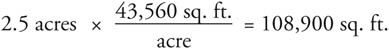2.   To get the total amount of product needed, multiply the area by the application rate.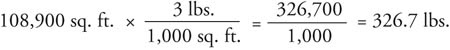After the rotary spreader is calibrated to apply the product at half the labeled rate, there are two ways to make the application.

Right Angle Method:  Make two passes at right angles to each other. Space passes at the effective swath width. For example, if the effective swath width is 8 feet, then after each pass, move the spreader over 8 feet from the center of the tire tracks to begin the next pass.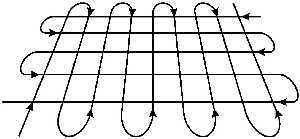Half-width Method:  Use parallel passes spaced apart at one-half the swath width. For example, if the swath width is 10 feet, make each pass at 5-foot intervals.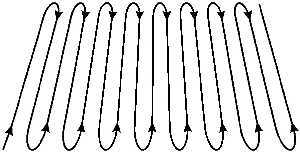Compiled by Wayne Buhler, PhD.